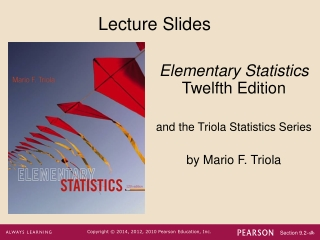DownloadDownload PresentationLecture Slides

# Lecture Slides

Télécharger la présentation## Lecture Slides

- - - - - - - - - - - - - - - - - - - - - - - - - - - E N D - - - - - - - - - - - - - - - - - - - - - - - - - - -
##### Presentation Transcript

1. Lecture Slides Elementary StatisticsTwelfth Edition and the Triola Statistics Series by Mario F. Triola

2. Chapter 9 Inferences from Two Samples 9-1 Review and Preview 9-2 Two Proportions 9-3 Two Means: Independent Samples 9-4 Two Dependent Samples (Matched Pairs) 9-5 Two Variances or Standard Deviations

3. Key Concept In this section we present methods for (1) testing a claim made about two population proportions and (2) constructing a confidence interval estimate of the difference between the two population proportions. This section is based on proportions, but we can use the same methods for dealing with probabilities or the decimal equivalents of percentages.

4. Notation for Two Proportions For population 1, we let: = population proportion = size of the sample = number of successes in the sample (the sample proportion) The corresponding notations apply to which come from population 2.

5. The pooled sample proportion is given by: Pooled Sample Proportion

6. Requirements 1. We have proportions from two independent simple random samples. 2. For each of the two samples, the number of successes is at least 5 and the number of failures is at least 5.

7. Test Statistic for Two Proportions For where (assumed in the null hypothesis)

8. Testing Two Proportions P-value: P-values are automatically provided by technology. If technology is not available, use Table A-2. Critical values: Use Table A-2. (Based on the significance level α, find critical values by using the procedures introduced in Section 8-2 in the text.)

9. Confidence Interval Estimate of p1 – p2

10. Example Do people having different spending habits depending on the type of money they have? 89 undergraduates were randomly assigned to two groups and were given a choice of keeping the money or buying gum or mints. The claim is that “money in large denominations is less likely to be spent relative to an equivalent amount in many smaller denominations”. Let’s test the claim at the 0.05 significance level.

11. Example Below are the sample data and summary statistics:

12. Example • Requirement Check: • The 89 subjects were randomly assigned to two groups, so we consider these independent random samples. • The subjects given the \$1 bill include 12 who spent it and 34 who did not. The subjects given the quarters include 27 who spent it and 16 who did not. All counts are above 5, so the requirements are all met.

13. Example Step 1: The claim that “money in large denominations is less likely to be spent” can be expressed as p1 < p2. Step 2: If p1 < p2 is false, then p1 ≥ p2. Step 3: The hypotheses can be written as:

14. Example Step 4: The significance level is α = 0.05. Step 5: We will use the normal distribution to run the test with:

15. Example Step 6: Calculate the value of the test statistic:

16. Example Step 6: This is a left-tailed test, so the P-value is the area to the left of the test statistic z = –3.49, or 0.0002. The critical value is also shown below.

17. Example Step 7: Because the P-value of 0.0002 is less than the significance level of α = 0.05, reject the null hypothesis. There is sufficient evidence to support the claim that people with money in large denominations are less likely to spend relative to people with money in smaller denominations. It should be noted that the subjects were all undergraduates and care should be taken before generalizing the results to the general population.

18. Example We can also construct a confidence interval to estimate the difference between the population proportions. Caution: The confidence interval uses standard deviations based on estimated values of the population proportions, and consequently, a conclusion based on a confidence interval might be different from a conclusion based on a hypothesis test.

19. Example Construct a 90% confidence interval estimate of the difference between the two population proportions. What does the result suggest about our claim about people spending large denominations relative to spending small denominations?

20. Example

21. Example The confidence interval limits do not include 0, implying that there is a significant difference between the two proportions. There does appear to be sufficient evidence to support the claim that “money in large denominations is less likely to be spent relative to an equivalent amount in many smaller denominations.”

22. Why Do the Procedures of This Section Work? The distribution of can be approximated by a normal distribution with mean p1, and standard deviation: The difference can be approximated by a normal distribution with mean p1 – p2and variance The variance of the differences between two independent random variables is the sum of their individual variances.

23. Why Do the Procedures of This Section Work? The preceding variance leads to We now know that the distribution of is approximately normal, with mean and standard deviation as shown above, so the z test statistic has the form given earlier.

24. Why Do the Procedures of This Section Work? When constructing the confidence interval estimate of the difference between two proportions, we don’t assume that the two proportions are equal, and we estimate the standard deviation as

25. Why Do the Procedures of This Section Work? In the test statistic use the positive and negative values of z (for two tails) and solve for The results are the limits of the confidence interval given earlier.